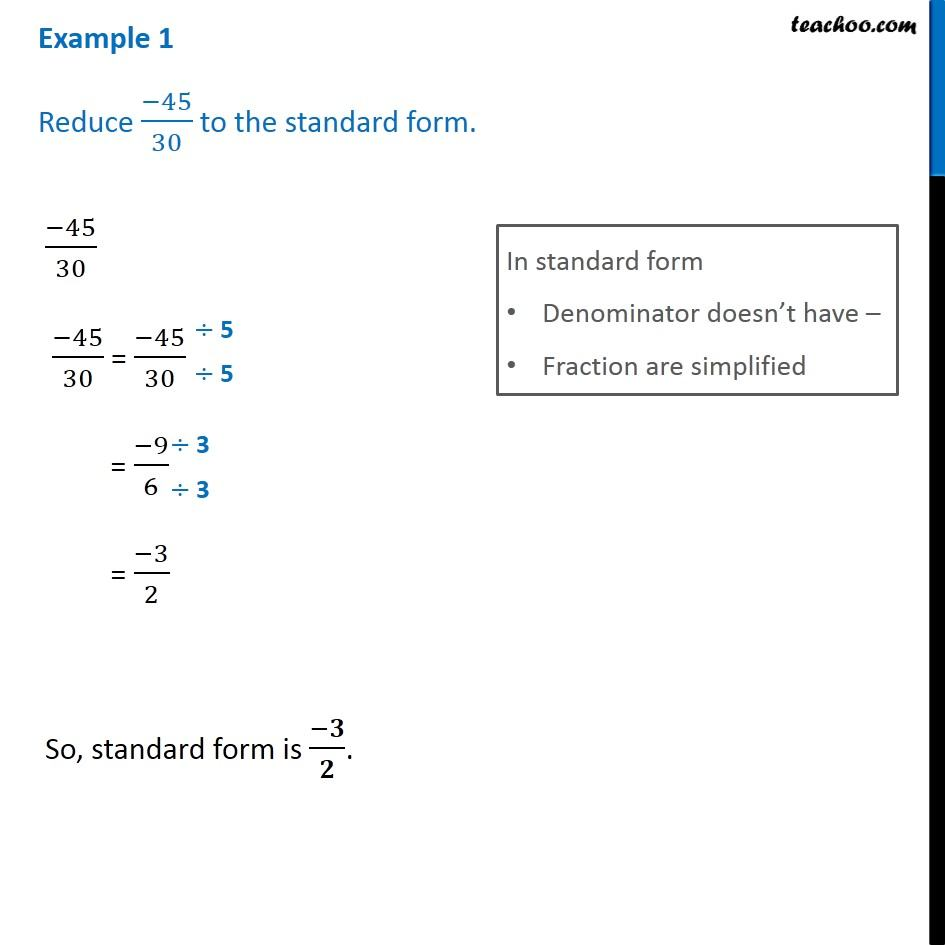Standard form of rational numbers

Chapter 9 Class 7 Rational Numbers
Concept wiseIntroducing your new favourite teacher - Teachoo Black, at only ₹83 per month

### Transcript

Example 1 Reduce (−45)/30 to the standard form. (−45)/30 (−45)/30 = (−45)/30 In standard form Denominator doesn’t have – Fraction are simplified = (−9)/6 = (−3)/2 So, standard form is (−𝟑)/𝟐.